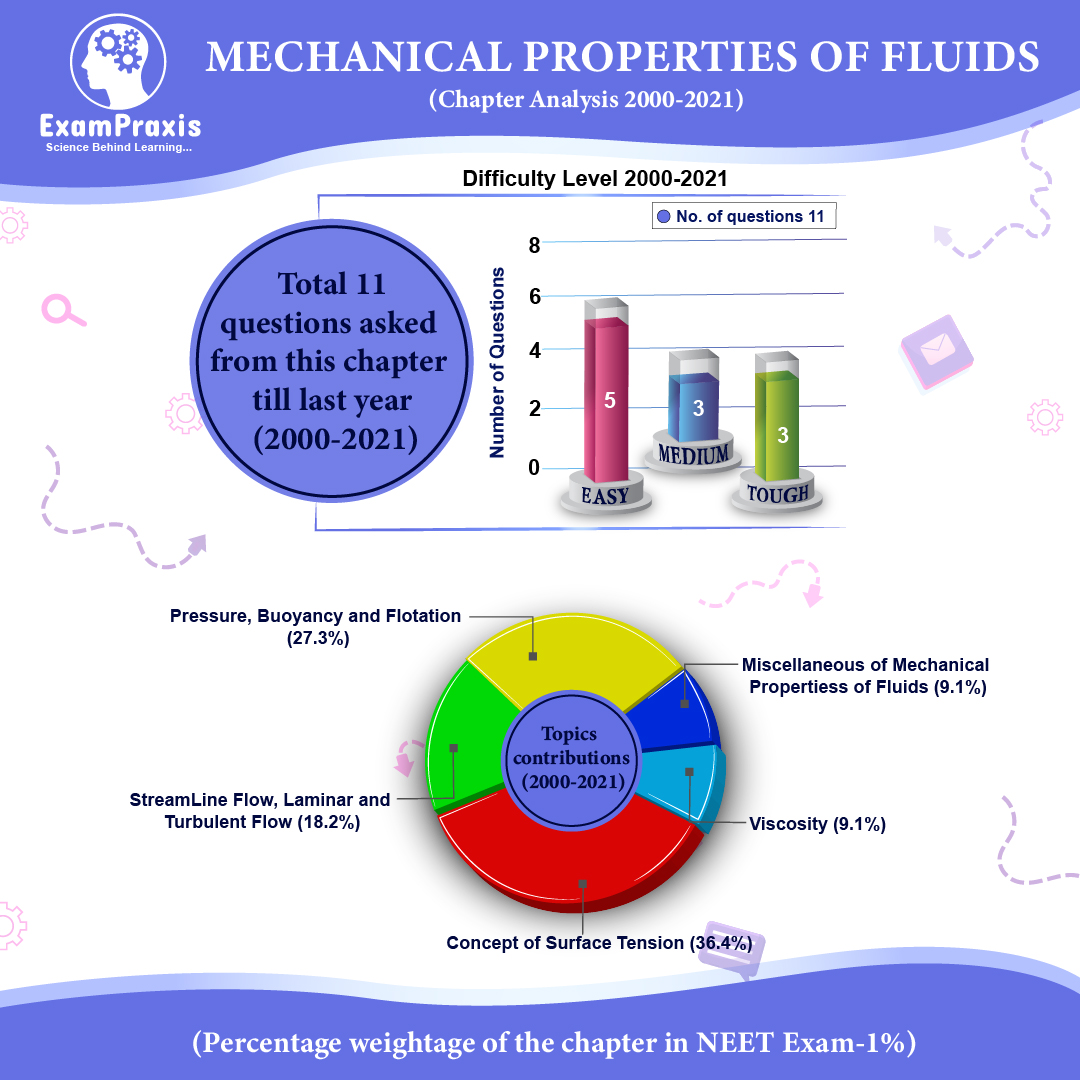• +91 141 4005482
• support@exampraxis.com

# Mechanical Properties of Fluids

#### View Study Materials Listing

What's in this Study Materials?

1. Pressure

• Average pressure is defined as the normal force acting per unit area
• ${{P}_{av}}=\frac{F}{A}$
• $P=\underset{\Delta A\to 0}{\mathop{\lim }}\,\left( \frac{\Delta F}{\Delta A} \right),$ It is a scalar quantity